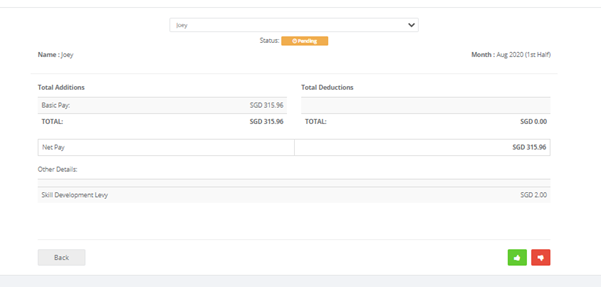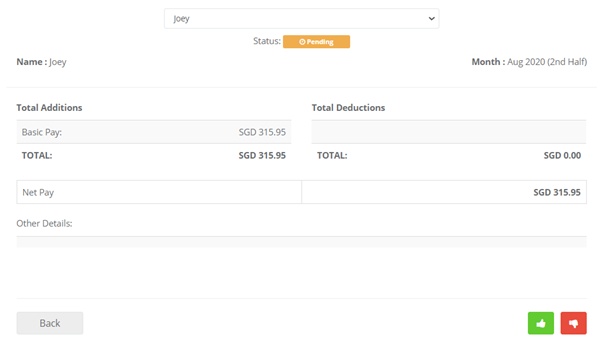# Introduction

This article will show how the system calculates the basic pay amount of the new/resigned employees if they are on a fortnightly pay period.

Scenario: The employee joined the company on the 14th of August 2020. The Basic Pay of \$1100 is effective from 14th of August 2020, and on a fortnightly pay period.

Number of working days in the month of August: 23.5

Workweek: 5.5 days

Total number of worked days in the month of August: 13.5

• Get the daily rate of the employee using the formula BasicPay / Number of working days in the month.

1100 / 23.5 = 46.81

• Using the daily rate, get the total value for the entire month following the formula Daily Rate * Total Number of worked days in the month.

46.81 * 13.5 = 631.91

• Divide the total value for the entire month into 2, and that’s how we compute for the fortnightly value.

631.91 / 2 = 315.96

Thus:

Fortnightly (first interval) = 315.96Fortnightly (second interval) = 315.95